Go back to  'Maths'

## Introduction to Addition and Subtraction

### Addition and subtraction are the most basic operations. These concepts are building blocks of understanding and operating on numbers. We add and subtract numbers, amounts and values in our everyday lives. Children are mechanically introduced to these concepts using numbers. However, this may not be fruitful in the long run. Mechanically rote memorizing the procedures increases the chances of silly mistakes.## What are addition and subtraction?

Addition can be visualised as 'putting together' or two or more quantities. It is important for children to see how when we put together two quantities it gives a larger group. For example, the image below shows, when the balls of the two groups were put together, it resulted in a third larger group.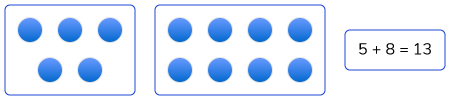Subtraction can be visualised as 'taking away'. For example the image below shows, there are 15 squares and we take away 4 of them. 11 would be remaining.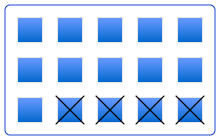Cuemath students learn to first visualise the idea of addition and subtraction using different visual models such as the number line, ten-frame, 20 frame. Once they are comfortable with visual models they move to numbers. Given below are images of a few visual models.

Use of real-life objects to show subtraction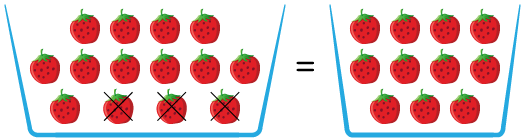Number line to show addition of numbers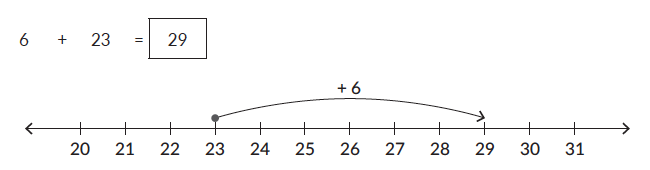## Topics closely related to Addition and Subtraction

The image shown below shows the topics one should be well versed with to understand addition & subtraction. It also shows the topics that these two operations are closely related to.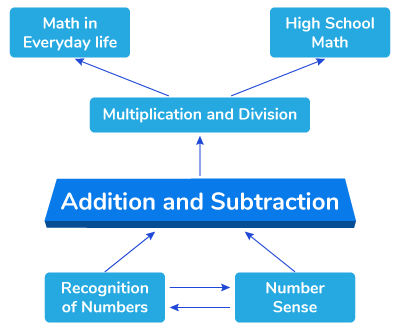## Here is how Cuemath students learn to visualise the addition of numbers

Experience this in a Cuemath class. Book a Cuemath demo class with a nearby centre

## How do you teach Addition and Subtraction?

1. Ask children to perform addition and subtraction using real-life objects like pens, pencils, chocolates, marbles.

2. Use math manipulatives like colour counters, abacus, flats, rods and blocks to help children perform these operations

3. Pop quiz: you can ask children 5 rapid-fire questions on addition and subtraction.

4. Based on the pocket money children receive Ask children to maintain a log of their expenses. At the end of the month, you can ask for the remaining amount.

5. Use flats, rods and blocks to show how the procedure works with larger numbers.